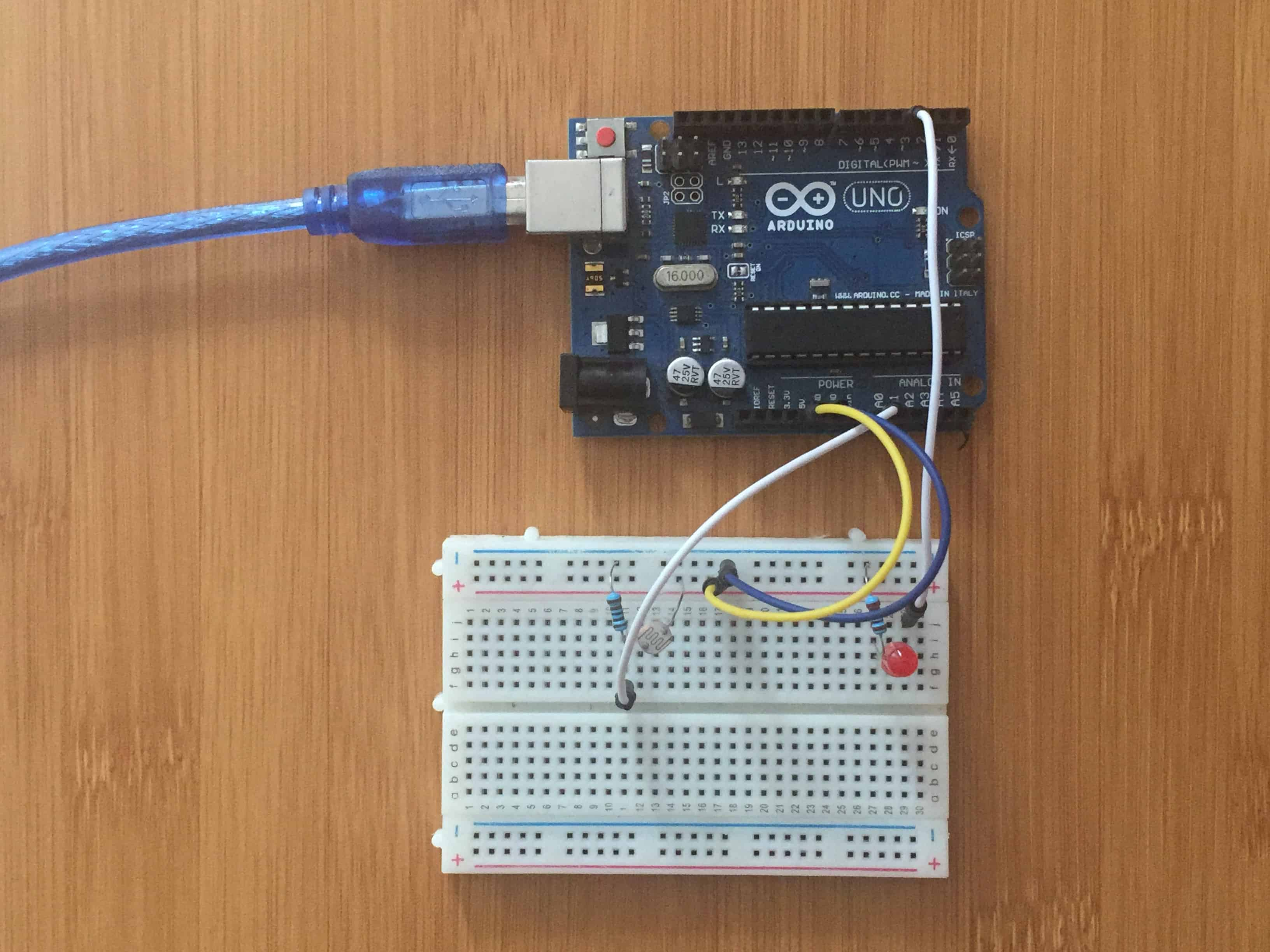# How to use LDR as a Light Sensor with Arduino.A light sensor with Arduino is a simple demonstration of how Arduino can be used in home automation, in this case controlling the lighting of bulbs depending on the amount of light in a given area.

In this project we are using a LDR (Light Dependent Resistor) to act as a photosensor so that when there is light the LED will turn off and when it gets dark the LED turns on automatically.

## What is a Light Dependent Resistor (LDR)?

An LDR is a component that has a (variable) resistance that changes with the light intensity that falls upon it. This allows them to be used in light sensing circuits.

The most common type of LDR has a resistance that falls with an increase in the light intensity falling upon the device.

LDRs are used mainly as sensors in for lighting switches to control light in homes and streets. They are also used as camera shutter controls. The LDR would be used to measure the light intensity which then adjusts the camera shutter speed to the appropriate level.

## Connecting the Light Sensor to Arduino

From the setup, we have a voltage divider cicuit using LDR and 100k resistor. The voltage divider output is fed to the analog pin A0 of the Arduino. The analog Pin senses the voltage and gives some analog value to Arduino.

The analog value changes according to the resistance of LDR. So, as the light falls on the LDR the resistance of it get decreased and hence the voltage value increase.

### Code for controlling LED with a Light sensor and Arduino.

``````int led=3; // led at pin3

void setup(){
pinMode(led,OUTPUT);
}
void loop(){